Courses

# Ex 5.2 NCERT Solutions- Arithmetic Progressions Class 10 Notes | EduRev

## Class 10 : Ex 5.2 NCERT Solutions- Arithmetic Progressions Class 10 Notes | EduRev

The document Ex 5.2 NCERT Solutions- Arithmetic Progressions Class 10 Notes | EduRev is a part of the Class 10 Course Mathematics (Maths) Class 10.
All you need of Class 10 at this link: Class 10

Ques 1: Fill in the blanks in the following table, given that ‘a’ is the first term, ‘d’ the common difference and an the nth term of the A.P.:

 a d n an (i) 7 3 8 … (ii) -18 … 10 0 (iii) … -3 18 -5 (iv) -18.9 2.5 … 3.6 (v) 3.5 0 105 …

Sol: (i) an = a + (n - 1) d
⇒ a8 = 7 + (8 - 1) 3
= 7 + 7 × 3
= 7 + 21
⇒ a8 = 28
(ii) an = a + (n - 1) d
⇒ a10 = - 18 + (10 - 1) d
⇒ 0 = - 18 + 9d
⇒ 9d = 18 ⇒ d = 18/9 = 2
∴ d = 2
(iii) an = a + (n - 1) d
⇒ - 5 = a + (18 - 1) × (- 3)
⇒ - 5 = a + 17 × (- 3)
⇒ - 5 = a - 51
⇒ a = - 5 + 51 = 46
Thus, a = 46
(iv) a= a + (n - 1) d
⇒ 3.6 = - 18.9 + (n - 1) × 2.5
⇒ (n - 1) × 2.5 = 3.6 + 18.9
⇒ (n - 1) × 2.5 = 22.5
⇒ n - 1 = 22.5/2.5 = 9
⇒ n = 9 + 1 = 10
Thus, n = 10
(v) an = a + (n - 1) d
⇒ an = 3.5 + (105 - 1) × 0
⇒ an = 3.5 + 104 × 0
⇒ an = 3.5 + 0 = 3.5
Thus, an = 3.5

Ques 2: Choose the correct choice in the following and justify:
(i) 30th term of the A.P.: 10, 7, 4, ...., is
(A) 97
(B) 77
(C) - 77
(D) - 87
(ii) 11th term of the A.P.: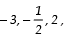, ...., is
(A) 28

(B) 22
(C) - 38
(D)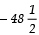Sol: (i) Here, a = 10, n = 30
∵ Tn = a + (n - 1) d and d = 7 - 10 = - 3
∴ T30 = 10 + (30 - 1) × (- 3)
⇒ T30 = 10 + 29 × (- 3)
⇒ T30 = 10 - 87 = - 77
Thus, the correct choice is (C) - 77.
(ii) Here, a = - 3, n = 11 and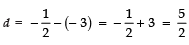∴Tn = a + (n - 1) d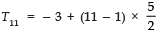⇒ T11 = - 3 + 25 = 22
Thus, the correct choice is (B) 22.

Ques 3: In the following A.Ps., find the missing terms in the boxes: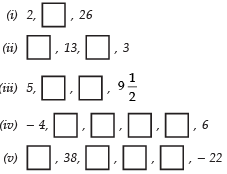Sol: (i) Here, a = 2, T3 = 26
Let common difference = d
∴ Tn = a + (n - 1) d
⇒ T3 = 2 + (3 - 1) d
⇒ 26 = 2 + 2d
⇒ 2d = 26 - 2 = 24
⇒ d = 24/2 = 12
∴ The missing term = a + d
= 2 + 12 =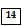(ii) Let the first term = a and common difference = d
Here, T2 = 13 and T4 = 3
T2 = a + d = 13
T4 = a + 3d = 3
∴T4 - T2 = (a + 3d) - (a + d) = 3 - 13
⇒ 2d = - 10
⇒ d = -10/2 = -5
Now, a + d = 13 ⇒ a + (- 5) = 13
⇒ a = 13 + 5 = 18
Thus, missing terms are a and a + 2d or 18 and 18 + (- 10) = 8
i.e., T1 =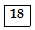and T3 =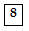(iii) Here, a = 5 and T4 =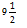since, T4 = a + 3d
⇒= 5 + 3d
⇒ 3d =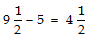⇒ d =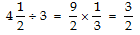∴ The missing terms are:
T2 = a + d =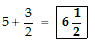T3 = a + 2d =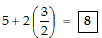(iv) Here, a = - 4 and T6 = 6
∵ Tn = a + (n - 1) d
∴T6 = - 4 + (6 - 1) d
⇒ 6 = - 4 + 5d
⇒ 5d = 6 + 4 = 10
⇒ d = 10¸ 5 = 2
∴T2 = a + d = - 4 + 2 = - 2
T3 = a + 2d = - 4 + 2 (2) = 0
T4 = a + 3d = - 4 + 3 (2) = 2
T5 = a + 4d = - 4 + 4 (2) = 4
∴ The missing terms are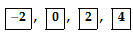(v) Here, T2 = 38 and T6 = - 22
∴T2 = a + d = 38
T6 = a + 5d = - 22
⇒ T6 - T2 = a + 5d - (a + d) = - 22 - 38
⇒ 4d = - 60
⇒ d = -60/4 = -15
∴ a + d = 38 ⇒ a + (- 15) = 38
⇒ a = 38 + 15 = 53
Now,
T3 = a + 2d = 53 + 2 (- 15) = 53 - 30 = 23
T4 = a + 3d = 53 + 3 (- 15) = 53 - 45 = 8
T5 = a + 4d = 53 + 4 (- 15) = 53 - 60 = - 7
Thus, the missing terms are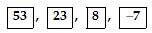Ques 4: Which term of the A.P.: 3, 8, 13, 18, ..., is 78?
Sol: Let the nth term = 78
Here, a = 3, ⇒ T1 = 3 and T2 = 8
∴ d = T2 - T1 = 8 - 3 = 5
Now, Tn = a + (n - 1) d
⇒ 78 = 3 + (n - 1) × 5
⇒ 78 - 3 = (n - 1) × 5
⇒ 75 = (n - 1) × 5
⇒ (n - 1) = 75¸ 5 = 15
⇒ n = 15 + 1 = 16
Thus, 78 is the 16th term of the given A.P.

Ques 5: Find the number of terms in each of the following A.Ps.:
(i) 7, 13, 19, ..., 205
(ii)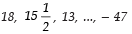Sol: (i) Here, a = 7
d = 13 - 7 = 6
Let the number of terms be n
∴Tn = 205
Now, Tn = a + (n - 1) × d
⇒ 7 + (n - 1) × 6 = 205
⇒ (n - 1) × 6 = 205 - 7 = 198
⇒ n - 1 = 198/6 = 33
∴n = 33 + 1 = 34
Thus, the required number of terms is 34.
(ii) Here, a = 18
d =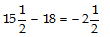Let the nth term = - 47
∴Tn = a + (n - 1) d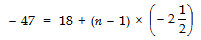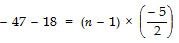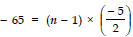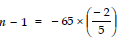⇒ n - 1 = (- 13) × (- 2) = 26
⇒ n = 26 + 1 = 27
Thus, the required number of terms is 27.

Ques 6: Check whether - 150 is a term of the A.P.: 11, 8, 5, 2 ...
Sol: For the given A.P., we have
a = 11
d = 8 - 11 = - 3
Let - 150 is the nth term of the given A.P.
∴ Tn = a + (n - 1) d
⇒ - 150 = 11 + (n - 1) × (- 3)
⇒ - 150 - 11 = (n - 1) × (- 3)
⇒ - 161 = (n - 1) × (- 3)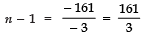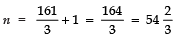But n should be a positive integer.
Thus, - 150 is not a term of the given A.P.

Ques 7: Find the 31st term of an A.P. whose 11th term is 38 and the 16th term is 73.
Sol: Here,
T31 = ?
T11 = 38
T16 = 73
If the first term = a and the common difference = d.
Then,
a + (11 - 1) d = 38
⇒ a + 10d = 38   ...(1)
and a + (16 - 1) d = 73
⇒ a + 15d = 73   ...(2)
Subtracting (1) from (2), we get
(a + 15d) - (a + 10d) = 73 - 38
⇒ 5d = 35
⇒ d = 35/5 = 7
From (1),
a + 10 (7) = 38
⇒ a + 70 = 38
⇒ a = 38 - 70 = - 32
∴T31 = - 32 + (31 - 1) × 7
⇒ T31 = - 32 + 30 × 7
⇒ T31 = - 32 + 210
⇒ T31 = 178
Thus, the 31st term is 178.

Ques 8: An A.P. consists of 50 terms of which 3rd term is 12 and the last term is 106. Find the 29th term.
Sol: Here, n = 50
T3 = 12
Tn = 106 ⇒ T50 = 106
If first term = a and the common difference = d
∴T3 = a + 2d = 12    ...(1)
T50 = a + 49d = 106    ...(2)
⇒ T50 - T3 ⇒ a + 49d - (a + 2d) = 106 - 12
⇒ 47d = 94
⇒ d = 94/47 = 2
From (1), we have
a + 2d = 12 ⇒ a + 2 (2) = 12
⇒ a = 12 - 4 = 8
Now, T29 = a + (29 - 1) d
= 8 + (28) × 2
= 8 + 56 = 64
Thus, the 29th term is 64.

Ques 9: If the 3rd and the 9th terms of an A.P. are 4 and - 8 respectively, which term of this A.P. is zero ?
Sol:
Here,
T3 = 4 and T9 = - 8
∴Using Tn = a + (n - 1) d
⇒ T3 = a + 2d = 4 ...(1)
T9 = a + 8d = - 8 ...(2)
Subtracting (1) from (2) we get
(a + 8d) - (a + 2d) = - 8 - 4
⇒ 6d = - 12
⇒ d = -12/6  = -2
Now, from (1), we have:
a + 2d = 4
⇒ a + 2 (- 2) = 4
⇒ a - 4 = 4
⇒ a = 4 + 4 = 8
Let the nth term of the A.P. be 0.
∴Tn = a + (n - 1) d = 0
⇒ 8 + (n - 1) × (- 2) = 0
⇒ (n - 1) × - 2 = - 8
⇒ n - 1 = -8/-2 = 4
⇒ n = 4 + 1 = 5
Thus, the 5th term of the A.P. is 0.

Ques 10: The 17th term of an A.P. exceeds its 10th term by 7. Find the common difference.
Sol: Let ‘a’ be the first term and ‘d’ be the common difference of the given A.P.
Now, using
Tn = a + (n - 1) d
T17 = a + 16d
T10 = a + 9d
According to the condition,
Tn + 7 = T17
⇒ (a + 9d) + 7 = a + 16d
⇒ a + 9d - a - 16d = - 7
⇒- 7d = - 7 ⇒ d = 1
Thus, the common difference is 1.

Ques 11: Which term of the A.P.: 3, 15, 27, 39, ... will be 132 more than its 54th term?
Sol: Here, a = 3
d = 15 - 3 = 12
Using Tn = a + (n - 1) d, we get
T54 = a + 53d
= 3 + 53 × 12
= 3 + 636 = 639
Let an be 132 more than its 54th term.
∴ an = T54 + 132
⇒ an = 639 + 132 = 771
Now an = a + (n - 1) d = 771
⇒ 3 + (n - 1) × 12 = 771
⇒ (n - 1) × 12 = 771 - 3 = 768
⇒ (n - 1) = 768/12 = 64
⇒ n = 64 + 1 = 65
Thus, 132 more than 54th term is the 65th term.

Ques 12: Two A.Ps. have the same common difference. The difference between their 100th terms is 100, what is the difference between their 1000th terms
Sol: Let for the 1st A.P., the first term =
∴T100 = a + 99d
And for the 2nd A.P., the first term = a'
∴  T'100= a' + 99d
According to the condition, we have:
= T100 -T'1000
⇒ a + 99d - (a' + 99d) = 100
⇒ a - a' = 100
Let, = T1000-T'1000
∴a + 999d - (a' + 999d) = x
⇒ a - a' = x ⇒ x = 100
∴The difference between the 1000th terms is 100.

Ques 13: How many three-digit numbers are divisible by 7?
Sol:  The first three digit number divisible by 7 is 105.
The last such three digit number is 994.
∴ The A.P. is 105, 112, 119, ....., 994
Here, a = 105 and d = 7
Let n be the required number of terms.
∴ Tn = a + (n - 1) d
⇒ 994 = 105 + (n - 1) × 7
⇒ (n - 1) × 7 = 994 - 105 = 889
⇒ (n - 1) = 889/7 = 147
⇒ n = 127 + 1 = 128
Thus, 128 numbers of 3-digit are divisible by 7.

Ques 14: How many multiples of 4 lie between 10 and 250?
Sol: ∵ The first multiple of 4 beyond 10 is 12.
The multiple of 4 just below 250 is 248.
∴ The A.P. is given by:
12, 16, 20, ....., 248
Here, a = 12 and d = 4
Let the number of terms = n
∴ Using Tn = a + (n - 1) d, we get
∴ Tn = 12 + (n - 1) × 4
⇒ 248 = 12 + (n - 1) × 4
⇒ (n - 1) × 4 = 248 - 12 = 236
⇒ n - 1 = 236/4 = 59
⇒ n = 59 + 1 = 60
Thus, the required number of terms = 60.

Ques 15: For what value of n, are the nth terms of two A.Ps.: 63, 65, 67, ... and 3, 10, 17, ... equal?
Sol: For the 1st A.P.
∵ a = 63 and d = 65 - 63 = 2
∴T= a + (n - 1) d
⇒ Tn = 63 + (n - 1) × 2
For the 2nd A.P.
∵ a = 3 and d = 10 - 3 = 7
∴ Tn = a + (n - 1) d
⇒ Tn = 3 + (n - 1) × 7
Now, according to the condition,
3 + (n - 1) × 7 = 63 + (n - 1) × 2
⇒ (n - 1) × 7 - (n - 1) × 2 = 63 - 3
⇒ 7n - 7 - 2n + 2 = 60
⇒ 5n - 5 = 60
⇒ 5n = 60 + 5 = 65
⇒ n = 65/5 = 13
Thus, the 13th terms of the two given A.Ps. are equal.

Ques 16: Determine the A.P. whose third term is 16 and the 7th term exceeds the 5th term by 12.
Sol: Let the first term = a and the common difference = d.
∴ Using Tn = a + (n - 1) d, we have:
T3 = a + 2d
⇒ a + 2d = 16             ...(1)
And T7 = a + 6d, T5 = a + 4d
According to the condition,
T7 - T5 = 12
⇒ (a + 6d) - (a + 4d) = 12
⇒ a + 6d - a - 4d = 12
⇒ 2d = 12
⇒ d = 12/2 = 6                 ...(2)
Now, from (1) and (2), we have:
a + 2 (6) = 16
⇒ a + 12 = 16
⇒ a = 16 - 12 = 4
∴The required A.P. is
4, [4 + 6], [4 + 2 (6)], [4 + 3 (6)], .....
or 4, 10, 16, 22, .....

Ques 17: Find the 20th term from the last term of the A.P.: 3, 8, 13, ..., 253.
Sol: We have, the last term l = 253
Here, d = 8 - 3 = 5
Since, the nth term before the last term is given by l - (n - 1) d,
∴We have
20th term from the end = l - (20 - 1) × 5
= 253 - 19 × 5
= 253 - 95 = 158

Ques 18: The sum of the 4th and 8th terms of an A.P. is 24 and the sum of the 6th and 10th terms is 44. Find the first three terms of the A.P.
Sol: Let the first term = a
And the common difference = d
∴ Using Tn = a + (n - 1) d,
T4 + T8 = 24
⇒ (a + 3d) + (a + 7d) = 24
⇒ 2a + 10d = 24
⇒ a + 5d = 12 ...(1)
And T6 + T10 = 44
⇒ (a + 5d) + (a + 9d) = 44
⇒ 2a + 14d = 44
⇒ a + 7d = 22 ...(2)
Now, subtracting (1) from (2), we get
(a + 7d) - (a + 5d) = 22 - 12
⇒ 2d = 10
⇒ d = 10/2 = 5
∴From (1), a + 5 × 5 = 12
⇒ a + 25 = 12
⇒ a = 12 - 25 = - 13
Now, the first three terms of the A.P. are given by:
a, (a + d), (a + 2d)
or - 13, (- 13 + 5), [- 13 + 2 (5)]
or - 13, - 8, - 3

Ques 19: Subba Rao started work in 1995 at an annual salary of ₹ 5000 and received an increment of ₹ 200 each year. In which year did his income reach ₹ 7000?
Sol: Here, a = ₹ 5000 and d = ₹ 200
Say, in the nth year he gets ₹ 7000.
∴ Using Tn = a + (n - 1) d, we get
7000 = 5000 + (n - 1) × 200
⇒ (n - 1) × 200 = 7000 - 5000 = 2000
⇒ n - 1 = 2000/200 = 10
⇒ n = 10 + 1 = 11
Thus, his income becomes ₹ 7000 in 11 years.

Ques 20: Ramkali saved ₹ 5 in the first week of a year and then increased weekly savings by ₹ 1.75. If in the nth week, her weekly savings become ₹ 20.75, find n.
Sol: Here, a = ₹ 5 and d = ₹ 1.75
∵ In the nth week her savings become ₹ 20.75.
∴Tn = ₹ 20.75
∴Using Tn = a + (n - 1) d, we have
20.75 = 5 + (n - 1) × (1.75)
⇒ (n - 1) × 1.75 = 20.75 - 5
⇒ (n - 1) × 1.75 = 15.75
⇒ n - 1 =15.75/1.75 = 9
⇒ n = 9 + 1 = 10
Thus, the required number of years = 10.

• Sum of First n Terms of an A.P.

(i) If the first term of an A.P. is ‘a’ and the common difference is ‘d’ then the sum of its first n terms is given by: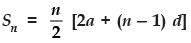(ii) If the last term of the A.P. is l then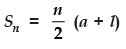Remember,
The sum of first n positive integers is given by: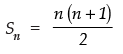Offer running on EduRev: Apply code STAYHOME200 to get INR 200 off on our premium plan EduRev Infinity!

## Mathematics (Maths) Class 10

178 videos|268 docs|103 tests

,

,

,

,

,

,

,

,

,

,

,

,

,

,

,

,

,

,

,

,

,

;